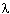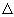# Physics QUESTIONSPanda

When the number of electrons striking the anode of an X-ray tube increases, the [removed]of the emitted X-rays increases.

When the speed of electrons striking the anode of an X-ray tube increases, the [removed]a0 of the emitted X-rays increases.

An X-ray tube emits X-rays with a wavelength of 1.00 x 10-11 m. Calculate the photon energy, in joules, that the emitted X-rays possess.

E = [removed]a0 x 10[removed]a1 joules

An X-ray tube emits X-rays with a wavelength of 1.00 x 10-11 m. Calculate the energy, in electron volts, that the X-rays possess.

[removed]a0 x 10[removed]a1 ev

An X-ray tube emits X-rays with a wavelength of 1.00 x 10-11 m. Determine the energy, in electron volts, possessed by the incident electrons.

[removed]a0 x 10[removed]a1 ev

An X-ray tube emits X-rays with a wavelength of 1.0 x 10-11 m. Calculate the potential that must be applied across the X-ray tube to give each incident electron its energy.

[removed]a0 x 10[removed]a1 ev

Calculate the highest frequency X-rays produced by 8.00 · 104 ev electrons.

[removed]a0 x 10[removed]a1 Hz

A television tube can accelerate electrons to 2.00 · 104 ev. Calculate the wavelength of emitted X-rays with the highest energy.= [removed]a0 x 10[removed]a1 m

Calculate the energy, in electron volts, of X-rays that have a frequency of 1.0 x 1019 Hz.

[removed]a0 x 10[removed]a1 ev

Calculate the de Broglie wavelength of a 5,100 kg truck traveling at 82 kph.= [removed]a0 x 10[removed]a1 m

Calculate the de Broglie wavelength of an electron traveling at 1.0 x 107 m/sec. (me = 9.1 · 10-31 kg).= [removed]a0 x 10[removed]a1 m

Calculate the approximate momentum change in a particle of mass 1.7 · 10-27 kg (a proton), initially at rest, whose position (x) is located to within 1.00 x 10-4 m.mv = [removed]a0 x 10[removed]a1 kg · m/sec.

Calculate the uncertainty of the velocity of a particle confined to a space of 1.0 x 10-9 m if the particle is an electron.

(me = 9.1 · 10-31 kg)v = [removed]a0 x 10[removed]a1 m/sec.

Calculate the uncertainty of the velocity of a particle confined to a space of 1.0 x 10-9 m if the particle is a proton.

(mp = 1.7 · 10-27 kg)v = [removed]a0 x 10[removed]a1 m/sec.

• 8 years ago
• 20
Answer(1)

Purchase the answer to view it

NOT RATED
•physics_x-rays.docx
•physics_x-rays.dot
Bids(1)